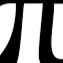Unresolved Detail In Plotted Equations

Sometimes the calculator detects that an equation is too complicated to plot perfectly in a reasonable amount of time. When this happens, the equation is plotted at lower resolution.

216 out of 241 found this helpful
Have more questions? Submit a request

•ANDREW TUCKER

\tan\left(\frac{\sin\left(x+\tan\left(y\right)\right)}{y!-\sin\left(x\right)}\right)=\tan\left(\frac{\tan\left(y+\sin\left(x\right)\right)}{x!-\tan\left(y\right)}\right)

\sin\left(x!^{\log\left(y!\right)}\right)=\tan\left(y^{\log\left(x\right)}\right)

y=\tan\left(\frac{\sin\left(\tan\left(\frac{\tan\left(\frac{\sin\left(\frac{\sin\left(\tan\left(x\right)\right)}{\cos\left(\sin\left(y\right)\right)}\right)}{y-\tan\left(x\right)}\right)}{\tan\left(\tan\left(\frac{\tan\left(\tan\left(x\right)\right)}{y}\right)\right)}\right)\right)}{\tan\left(\tan\left(\tan\left(\frac{\tan\left(\sin\left(x\right)\right)}{y+\tan\left(x\right)}\right)\right)\right)}\right)

y=\tan\left(\frac{\tan\left(\frac{\sin\left(x+\tan\left(x\right)\right)}{y}\right)}{\sin\left(\tan\left(y\right)\right)}\right)

•Ianskot492

Thank you all for making it easier to break my computer!

•Grhumphrey20
Edited by Grhumphrey20
•Tanksear Industries
•First Last

Another time the fine detail is unresolved: tan(x/y)=cot(y/x)

•Leo s.

\sin\left(y-x!\right)\ge\cos\left(x-y!\right)

inverse=

\sin\left(y-x!\right)\le\cos\left(x-y!\right)

•Christopher Hart

cos x^y+sin y^x=cos xy+sin xy

•Fanyi Zhao

tan(x+y)+tan(x-y)=1

•Lking22

\tan\left(x\right)=\cot\left(\frac{y}{\left|x\right|}\right)

•Owen Korver

sin(cos(tan(csc(sec(cot(log(x)))))))=sin(cos(tan(csc(sec(cot(log(y)))))))

•Melanie Green

I like combining these!

this one doesn't work well:

y=7\sin(6xy)

•Aidean The Awesome

sin(cos(x))=(cos(sin(y)))/tan(xy) isn't exactly crazy, but desmos will say that it cannot fully resolve it anyway.

•Owen Korver

tan(x)>cos(y)

Trig functions take things to weird levels

•Leo s.

\sin\left(y^{y^{\tan\left(23^{x^x}\right)}}\right)=\cot\left(y^{y^{y^{\sin\left(x\right)}}}\right)

looks kind of like this :

U

""

it does!

•Zoe Griffith

y!=cos(x!)

Laggy, but you will not be disappointed.

•Dmytro Hlukhaniuk

That`s not the case with unresolved pattern or detail, but looks cool!

\left(x-1y\right)\left(x+1\right)\left(xy-2y\right)\left(yx-3\right)\left(xy^2-2\right)=2

•Kerndr

and sin(xy)=sin(y)+sin(x) is cool

•3142 maple

\tan\left(y\cos\left(x\right)\right)=\tan\left(x\cos y\right)

Beautiful graph.

•25jw0157

I thank your some of your functions and equations to create this: https://www.desmos.com/calculator/frfgddorer

•D35M05BR34K4G3MA5T3R

BTW, none of the non-functions are showing, so show the ones you want to see.

•Lie

This one is laggy so beware! \frac{x}{y}=\frac{3}{\sec\left(x^{5^y}\right)}

•Creatorext

try \operatorname{floor}\left(yx\right)=xy^2

•. 狼丸

https://www.desmos.com/calculator/p2yid2mrzz
I think the plotter hates me now.

•Computingpotatochips

Try altering c to change adjustable fine detail. https://www.desmos.com/calculator/hhaqt3tpxr

•None

This doesn’t have unresolved detail, but it is awesome.

https://www.desmos.com/calculator/e24amhkpjl

•Matthew Ford

y=\csc\left(\frac{xy}{\sin\left(x\right)}\right) try this

•Tanksear Industries

\left(\tan\left(x\right)\right)^{\left(\sec\left(x\right)\right)^{\sin x}}\le\cot\left(\csc\left(\cos\left(y\right)\right)\right)

Sideways grass

•Jonahscott2020

\ln\left(e^x\right)=x and \ln\left(e^y\right)=y are fun.

•Lking22

\frac{\left|\operatorname{arcsec}x\right|}{\cot y}=\cos\left(\frac{x}{y}\left|x\right|\right)x

UwU

•Jonahscott2020

https://www.desmos.com/calculator/1sqbogyylx  Time to play some Factored checkers or Graphed chess